数组的排序 原

u      数组的排序

l      内部排序

(1) 交换式排序法

冒泡法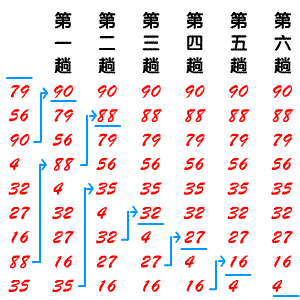<?php

//简单的

\$arr=array(0,5,-1);
//这是一个中间变量
\$temp=0;
//我们要把数组，从小到大

//外层循环
for(\$i=0;\$i<count(\$arr)-1;\$i++){

for(\$j=0;\$j<count(\$arr)-1-\$i;\$j++){

//说明前面的数比后面的数大,就要交换

if(\$arr[\$j]>\$arr[\$j+1]){

\$temp=\$arr[\$j];
\$arr[\$j]=\$arr[\$j+1];
\$arr[\$j+1]=\$temp;

}
}

}

//输出
print_r(\$arr);

?>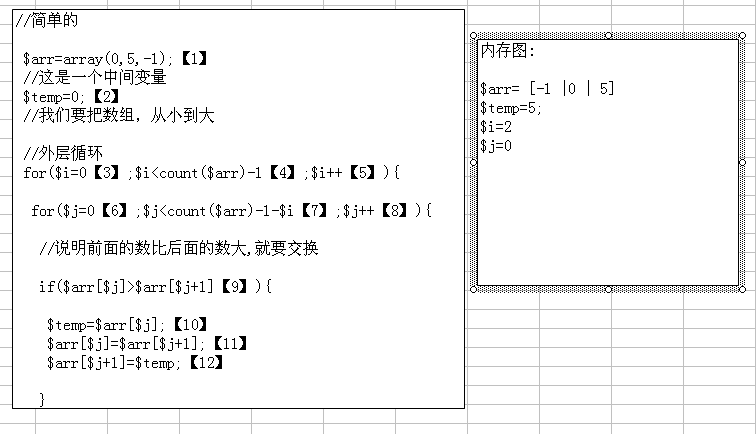<?php

//简单的

//现在我们把冒泡法封装成函数，利用以后使用

function bubbleSort(&\$myarr){

//这是一个中间变量

\$temp=0;

//我们要把数组，从小到大

//外层循环

for(\$i=0;\$i<count(\$myarr)-1;\$i++){

for(\$j=0;\$j<count(\$myarr)-1-\$i;\$j++){

//说明前面的数比后面的数大,就要交换

if(\$myarr[\$j]>\$myarr[\$j+1]){

\$temp=\$myarr[\$j];

\$myarr[\$j]=\$myarr[\$j+1];

\$myarr[\$j+1]=\$temp;

}

}

}

echo "<br/>函数中的myarr数组";

print_r(\$myarr);

}

\$arr=array(0,5,-1);

//使用函数去排序

bubbleSort(\$arr);

//输出

print_r(\$arr);

?>

* 数组默认传递的是值，不是地址

快速排序法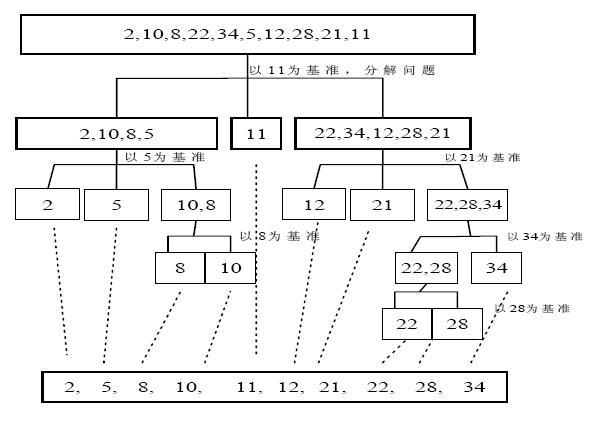选择排序法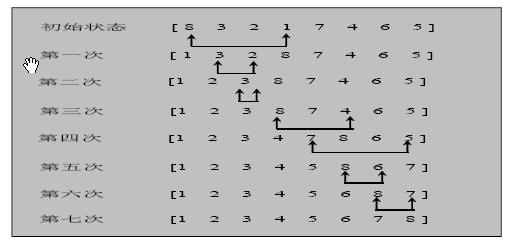function selectSort(&\$arr){

\$temp=0;

for(\$i=0;\$i<count(\$arr)-1;\$i++){

//假设 \$i就是最小的数

\$minVal=\$arr[\$i];

//记录我认为的最小数的下标

\$minIndex=\$i;

for(\$j=\$i+1;\$j<count(\$arr);\$j++){

//说明我们认为的最小值，不是最小

if(\$minVal>\$arr[\$j]){

\$minVal=\$arr[\$j];

\$minIndex=\$j;

}

}

//最后交换

\$temp=\$arr[\$i];

\$arr[\$i]=\$arr[\$minIndex];

\$arr[\$minIndex]=\$temp;

}

}

插入排序法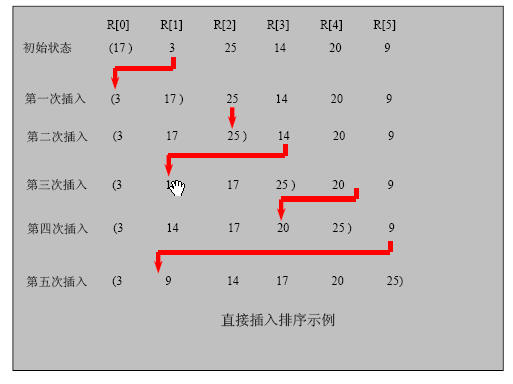//插入排序法(->)

function insertSort(&\$arr){

//先默认下标为0 这个数已经是有序

for(\$i=1;\$i<count(\$arr);\$i++){

//\$insertVal是准备插入的数

\$insertVal=\$arr[\$i];

//准备先和\$insertIndex比较

\$insertIndex=\$i-1;

//如果这个条件满足，说明，我们还没有找到适当的位置

while(\$insertIndex>=0&&\$insertVal<\$arr[\$insertIndex]){

//同时把数后移

\$arr[\$insertIndex+1]=\$arr[\$insertIndex];

\$insertIndex--;

}

//插入(这时就给\$insertVal找到适当位置)

\$arr[\$insertIndex+1]=\$insertVal;

}

}

l      外边排序

u      查找

顺序查找

//要求从一个数组 \$arr=array(46,90,900,0,-1)中查找一个 34,如果查找则输出该数//的下标，如果找不到，则输出，查无此数

\$arr=array(46,90,900,0,-1,-1);

function search(&\$arr,\$findVal){

\$flag=false;

for(\$i=0;\$i<count(\$arr);\$i++){

if(\$findVal==\$arr[\$i]){

echo "找到了，下标为=\$i";

\$flag=true;

//break;

}

}

if(!\$flag){

echo '查询不到';

}

}

search(\$arr,-1);

二分查找法（必须掌握）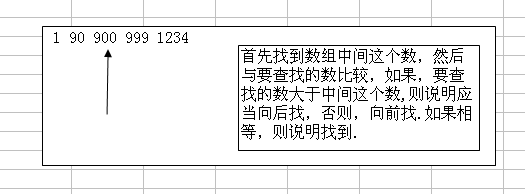//二分查找函数

function binarySearch(&\$arr,\$findVal,\$leftIndex,\$rightIndex){

// \$rightIndex>\$leftIndex 说明没有数

if(\$rightIndex<\$leftIndex){

echo "找不到该数";

return ;

}

//找到中间这个数

\$middleIndex=round((\$rightIndex+\$leftIndex)/2);

//如果大于则，向后面找

if(\$findVal>\$arr[\$middleIndex]){

binarySearch(\$arr,\$findVal,\$middleIndex+1,\$rightIndex);

}

//如果是小于中间这个数，则向前面找

else if(\$findVal<\$arr[\$middleIndex]){

binarySearch(\$arr,\$findVal,\$leftIndex,\$middleIndex-1);

}else{

echo "找到这个数 下标是 \$middleIndex";

}

}

u      多维数组-二维数组

\$arr=array( array(1,2,3), array(5,6,9) );

\$arr=array(1,0);

二维数组在内存中存在的形式: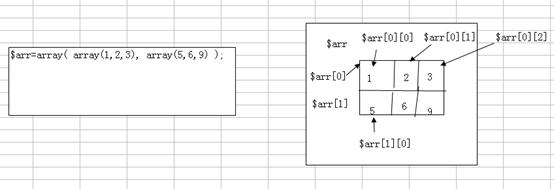0 0 0 0 0 0

0 0 1 0 0 0

0 2 0 3 0 0

0 0 0 0 0 0

//定义一个二维数组

\$arr=array(

array(0,0,0,0,0,0,1),

array(0, 0, 1, 0 ,0 ,0,5),

array(0 ,2 ,0, 3, 0, 0,90),

array(0,0,0,0,0,0,100));

//遍历二维数组()

for(\$i=0;\$i<count(\$arr);\$i++){

//遍历每行的数据

for(\$j=0;\$j<count(\$arr[\$i]);\$j++){

echo \$arr[\$i][\$j]."&nbsp;";

}

echo "<br/>";

}

echo "<br/>".\$arr;

1．  打印如下图型：

*********

*********

*********

*********

*********

*********

2．  打印如下图型：

*

**

***

****

*****

3．  打印如下图型：

2.       空心金字塔

3.       空心菱形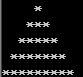function printTest(\$n,\$name){

}

view.php

<html>

<meta http-equiv="content-type" content="text/html;charset=utf-8"/>

<form action="homework01.php" method="post">

<select name="shape">

<option value="a">矩形</option>

<option value="b">半金字塔</option>

<option value="c">金字塔</option>

<option value="d">金字塔</option>

<option value="e">金字塔</option>

</select><br/>

<input type="submit" value="打印"/>

</form>

</html>

homework01.php

<?php

//死去活来

//\$n表示层数

\$n=\$_REQUEST['layer'];

//"a"->表示矩形 "b"=>一半金子塔 "c"=>完整的金字塔

\$shape=\$_REQUEST['shape'];

\$starNum=5;

//swtich

function printShape(\$n,\$shape,\$starNum=5){

switch(\$shape){

case "a":

for(\$i=1;\$i<=\$n;\$i++){

for(\$j=1;\$j<=\$starNum;\$j++){

echo "*";

}

//打完一层后，换行

echo "<br/>";

}

break;

case "b":

for(\$i=1;\$i<=\$n;\$i++){

for(\$j=1;\$j<=\$i;\$j++){

echo "*";

}

echo "<br/>";

}

break;

case "c":

break;

}

}

//调用

printShape(\$n,\$shape);

?>

1.      已知手掌游戏机一台320元，mp3一台260元。

<?php

\$gamerPrice=320;

\$mp3Price=260;

\$gamerNum=5;

\$mp3Num=13;

\$totalPrice=\$gamerPrice*\$gamerNum+ \$mp3Price* \$mp3Num;

\$avgPrice= \$totalPrice/ 18;

?>

1.      跳水比赛，8个评委打分。运动员的成绩是8个成绩取掉一个最高分，去掉一个最低分，剩下的6个分数的平均分就是最后得分。使用一维数组实现打分功能。

<?php

\$arr=array(90,23,89,100,2,5,89,44);

//这里有一个思路，如果只想知道最低分和最高分是多少，则可以排序.

//找出给出最低分的裁判

//该函数可以返回给出最低分的裁判的下标

function findMin(\$arr){

//假设第一个人就是给出最低分的裁判

\$minFen=\$arr;

\$minIndex=0;

for(\$i=1;\$i<count(\$arr);\$i++){

//如果下面条件成立，说明\$i 裁判给出的成绩更低

if(\$minFen>\$arr[\$i]){

\$minFe=\$arr[\$i];

\$minIndex=\$i;

}

}

return    \$minIndex;

}

?>

u      php面向对象编程

<?php

\$cat1_name=”小白”;

\$cat1_age=3;

\$cat1_color=”白色”;

\$cat2_name=”小花”;

\$cat2_age=100;

\$cat2_color=”花色”;

?>

<?php

class Cat{

public \$name;

public \$age;

public \$color;

}

//创建一只猫

\$cat1=new Cat();

\$cat1->name="小白";

\$cat1->age=3;

\$cat1->color="白色";

\$cat2=new Cat();

\$cat2->name="小花";

\$cat2->age=100;

\$cat2->color="颜色";

//如果我们找到一只猫,只要找到 \$cat1, 那么该变量所有相关的属性都通通的找到

\$findCatName="小花";

if(\$cat2->name==\$findCatName){

echo \$cat2->name."||".\$cat2->age."||".\$cat2->color;

}

?>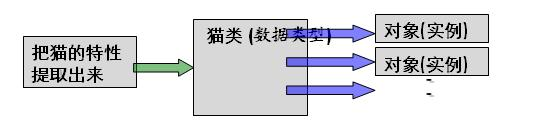类是抽象的，代表一类事物

对象是具体，是类的一个具体实例

类是对象的模板, 对象是类的一个个体实例

02/18
0
0
PHP array_multisort—对多个数组或多维数组进行排序

PHP中array_multisort可以用来一次对多个数组进行排序，或者根据某一维或多维对多维数组进行排序。 关联（string）键名保持不变，但数字键名会被重新索引。 输入数组被当成一个表的列并以行来...

2017/02/05
0
0
PHP数组常用函数分类整理

2016/07/05
45
0
PHP 数组函数-数组排序

php数组排序函数 sort ( &\$arr [,fruits] ) 对数组进行从低到高排序 ，并赋予新的键名 返回bool rsort ( &\$arr [,fruits] ) 对数组进行逆向排序 并赋予新的键名 asort ( &\$arr [,fruits] ) ...

xinson
2014/11/03
25
0

2011/07/23
9.7K
2

29分钟前
7
0
OSChina 周四乱弹 —— 我气的脸都黑了！

Osc乱弹歌单（2019）请戳（这里） 【今日歌曲】 小小编辑推荐《Red Battle》- 高橋李依 / 豊崎愛生 《Red Battle》- 高橋李依 / 豊崎愛生 手机党少年们想听歌，请使劲儿戳（这里） @丶Lion ...

42分钟前
554
22

https://www.bilibili.com/video/av64849038?from=search&seid=11632913960900279653

5
0

[TOC] 1.1.1什么是组件 一个vue文件就是一个组件 组件将html标签/css样式/对应JS打包成一个整体，也可以理解钻进一个具有样式和特效的自定义标签。 一、编写组件（提供方）<template> <di...

5
0

JAVA日知录

9
0